# Relational plots in Seaborn – Part II

• Difficulty Level : Easy
• Last Updated : 29 Jul, 2021

Prerequisite: Relational Plots in Seaborn – Part I
In the previous part of this article, we learnt about the relplot(). Now, we will be reading about the other two relational plots, namely scatterplot() and lineplot() provided in seaborn library. Both these plots can also be drawn with the help of kind parameter in relplot(). Basically relplot(), by default, gives us scatterplot() only, and if we pass the parameter kind = “line”, it gives us lineplot().

Example 1: Using relplot() to visualize tips dataset

## Python3

 `import` `seaborn as sns` `sns.``set``(style ``=``"ticks"``)` ` `  `tips ``=` `sns.load_dataset(``'tips'``)` `sns.relplot(x ``=``"total_bill"``, y ``=``"tip"``, data ``=` `tips)`

Output :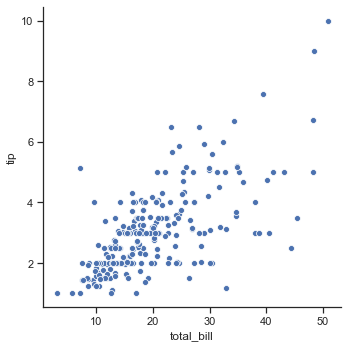Example 2: Using relplot() with kind=”scatter”.

## Python3

 `import` `seaborn as sns`     `sns.``set``(style ``=``"ticks"``) ` `tips ``=` `sns.load_dataset(``'tips'``)`   `sns.relplot(x ``=``"total_bill"``,` `            ``y ``=``"tip"``,` `            ``kind ``=``"scatter"``, ` `            ``data ``=` `tips)`

Output :Example 3: Using relplot() with kind=”line”.

## Python3

 `import` `seaborn as sns`     `sns.``set``(style ``=``"ticks"``) ` `tips ``=` `sns.load_dataset(``'tips'``)`   `sns.relplot(x ``=``"total_bill"``,` `            ``y ``=``"tip"``,` `            ``kind ``=``"line"``,` `            ``data ``=` `tips)`

Output :Though both these plots can be drawn using relplot(), seaborn also have separate functions for visualizing these kind of plots. These functions do provides some other functionalities too, compared to relplot(). Let us discuss about these function in more detail:

## Seaborn.scatterplot()

The scatter plot is a mainstay of statistical visualization. It depicts the joint distribution of two variables using a cloud of points, where each point represents an observation in the dataset. This depiction allows the eye to infer a substantial amount of information about whether there is any meaningful relationship between them.

Syntax :

`seaborn.scatterplot(x=None, y=None, data=None, **kwargs)`

Parameters :

Example 1: Plotting a scatterplot using marker to differentiate between timing of the people visiting the restaurant.

## Python3

 `import` `seaborn as sns`     `sns.``set``(style ``=``"ticks"``) ` `tips ``=` `sns.load_dataset(``'tips'``)` `markers ``=` `{``"Lunch"``: ``"s"``, ``"Dinner"``: ``"X"``}`   `ax ``=` `sns.scatterplot(x ``=``"total_bill"``,` `                     ``y ``=``"tip"``, ` `                     ``style ``=``"time"``,` `                     ``markers ``=` `markers,` `                     ``data ``=` `tips)`

Output: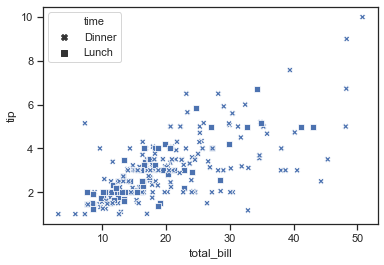Example 2: Passing data vectors instead of names in a data frame.

## Python3

 `import` `seaborn as sns`     `iris ``=` `sns.load_dataset(``"iris"``)`   `sns.scatterplot(x ``=` `iris.sepal_length,` `                ``y ``=` `iris.sepal_width,` `                ``hue ``=` `iris.species,` `                ``style ``=` `iris.species)`

Output: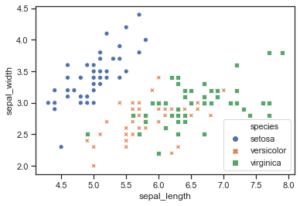## Seaborn.lineplot()

Scatter plots are highly effective, but there is no universally optimal type of visualization. For certain datasets, you may want to consider changes as a function of time in one variable, or as a similarly continuous variable. In this case, drawing a line-plot is a better option.

Syntax :

`seaborn.lineplot(x=None, y=None, data=None, **kwargs)`

Parameters :

Example 1: Basic visualization of “fmri” dataset using lineplot()

## Python3

 `import` `seaborn as sns`     `sns.``set``(style ``=` `'whitegrid'``)` `fmri ``=` `sns.load_dataset(``"fmri"``)`   `sns.lineplot(x ``=``"timepoint"``,` `             ``y ``=``"signal"``,` `             ``data ``=` `fmri)`

Output :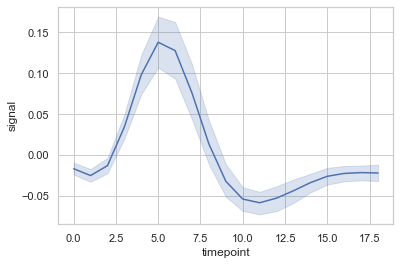Example 2: Grouping data points on the basis of category, here as region and event.

## Python3

 `import` `seaborn as sns`     `sns.``set``(style ``=` `'whitegrid'``)` `fmri ``=` `sns.load_dataset(``"fmri"``)`   `sns.lineplot(x ``=``"timepoint"``,` `             ``y ``=``"signal"``,` `             ``hue ``=``"region"``,` `             ``style ``=``"event"``,` `             ``data ``=` `fmri)`

Output :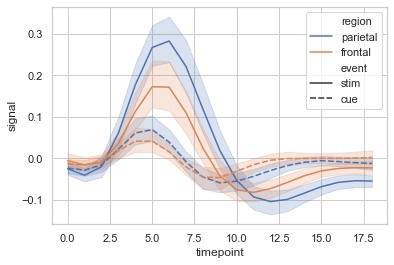Example 3: A complex plot visualizing “dots” dataset, to show the power of seaborn. Here, in this example, quantitative color mapping is used.

## Python3

 `import` `seaborn as sns`     `sns.``set``(style ``=` `'whitegrid'``)` `dots ``=` `sns.load_dataset(``"dots"``).query(``"align == 'dots'"``)`   `sns.lineplot(x ``=``"time"``, ` `             ``y ``=``"firing_rate"``,` `             ``hue ``=``"coherence"``,` `             ``style ``=``"choice"``,` `             ``data ``=` `dots)`

Output :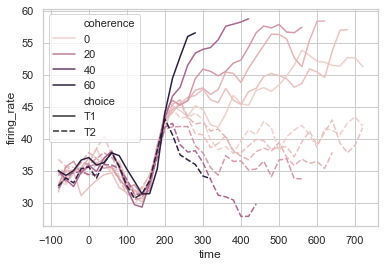My Personal Notes arrow_drop_up
Recommended Articles
Page :